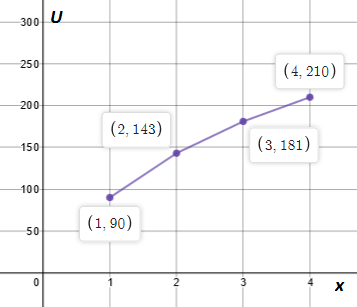# Utility is a type of function that occurs in economics. When a consumer receives x-units of a ...

## Question:

Utility is a type of function that occurs in economics. When a consumer receives x-units of a certain product, a certain amount of pleasure, or utility U, is derived.

The following is a graph of a utility function.

Find the average rate of change of each portion of U as x changes from 0 to 1; from 1 to 2, from 2 to 3, from 3 to 4.## Average Rate of Change

The average rate of change of a function is equal to the slope between two points on that function. Thus, we can only find the average rate of change if we're examining an interval, since we need two points in order to calculate it.

{eq}\frac{f(b) - f(a)}{b-a} {/eq}

Become a Study.com member to unlock this answer!

We've been asked to find four average rates of change. However, we are unable to find the first, as this function is not defined for us over the...Average Rate of Change: Definition, Formula & Examples

from

Chapter 20 / Lesson 5
45K

Learn to define the average rate of change. Discover the average rate of change formula. Learn how to find the average rate of change over an interval.